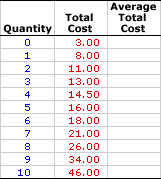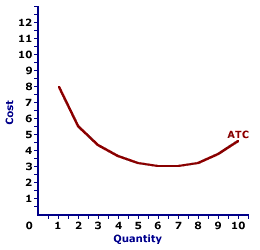Tuesday  June 6, 2023
 AmosWEB means Economics with a Touch of Whimsy!SLOPE, SHORT-RUN AGGREGATE SUPPLY CURVE: The short-run aggregate supply (SRAS) curve has a positive slope, reflecting the direct relation between the price level and aggregate real production. A higher price level is related to more real production and a lower price level is related to less real production. The general reason is similar to that of market supply curves--the opportunity cost of production--three specific reasons can be identified: (1) inflexible resource prices that often makes it easier to reduce aggregate real production and resource employment when the price level falls; (2) the pool of natural unemployment, consisting of frictional and structural unemployment, that can be used temporarily to increase aggregate real production when the price level rises; and (3) imbalances in the purchasing power of resource prices that can temporarily entice resource owners to produce more or less aggregate real production than the would at full employment.AVERAGE TOTAL COST:

Total cost per unit of output, found by dividing total cost by the quantity of output. When compared with price (per unit revenue), average total cost (ATC) indicates the per unit profitability of a profit-maximizing firm. Average total cost is one of three average cost concepts important to short-run production analysis. The other two are average fixed cost and average variable cost. A related concept is marginal cost.
Average total cost is the total cost per unit of output incurred when a firm engages in short-run production. It can be found in two ways. Because average total cost is total cost per unit of output, it can be found by dividing total cost by the quantity of output. Alternatively, because total cost is the sum of total variable cost and total fixed cost, average total cost can be derived by summing average variable cost and average fixed cost.

In general, average total cost decreases with additional production at relatively small quantities of output, then eventually increases with relatively large quantities of output. This pattern is illustrated by a U-shaped average total cost curve.

Average total cost, when combined with price, determines per unit profit or loss that a profit-maximizing firm receives from short-run production. If price is greater than average total cost, then the firm receives positive economic profit per unit. If price is less than average total cost, the firm incurs a loss, or negative economic profit, per unit. If price is equal to average total cost, then the firm is just breaking even, receiving neither a per unit profit nor incurring a per unit loss.

### Calculating Average Total Cost

The standard method of calculating average total cost is to divide total cost by the quantity, illustrated by this equation:
 average total cost = total costquantity of output
An alternative specification for average total cost is found by summing average variable cost and average fixed cost:
 average total cost = average variable cost + average fixed cost
An alternative equation computes total cost from average total cost:
 total cost = average total cost x quantity of output

### Stuffed Animal Cost

Average Total CostInsight into average total cost can be had using the table to the right, which presents the total cost of producing Wacky Willy Stuffed Amigos. The first column is the quantity of Stuffed Amigos rolling off the assembly line, ranging from 0 to 10. The second column is the total cost of producing each quantity, ranging from \$3 to \$46. If 5 Stuffed Amigos are produced, then the total cost incurred in their production is \$16. The production of 9 Stuffed Amigos, in comparison, incurs a total cost of \$34.

Deriving average total cost is as simple as dividing the second column of total cost values by the first column of output quantity values. The average total cost of producing 1 Stuffed Amigo is relatively easy--divide \$8 by 1 Stuffed Amigo. If the result of this calculation is not readily obvious, click the [One] button.

The average total cost of two Stuffed Amigos might be a little less obvious, but not much. Click the [Two] button to reveal that the average total cost is \$5.50, which is \$11 divided by 2 Stuffed Amigos. A click of the [Three] shows that the average total cost of producing 3 Stuffed Amigos is \$4.33, \$13 divided by 3. The remaining average total cost values can be displayed by clicking the [Remaining] button.

With all numbers displayed, what interesting insight into average total cost can be had?

• First, average total cost is relatively high for the first Stuffed Amigo produced, then declines. However, it reaches a low, then rises with production of the last few Stuffed Amigos.

• Second, average total cost remains positive, it never reaches a zero value and never turns negative. The only way for negative average total cost is for negative total cost, which makes no theoretical or practical sense.

• Third, the average total cost values in this table are comprised of two parts--average variable cost and average fixed cost. In other words, the \$4.60 average total cost of producing 10 Stuffed Amigos can be broken down into something like \$4.30 of average variable cost and \$0.30 of average fixed cost.

### The Average Total Cost Curve

Average Total Cost CurveThe relation between average total cost and the quantity of production can be represented by a curve, such as the one conveniently presented in the exhibit to the right.

The key feature of this average total cost is the shape. It is U-shaped, meaning it has a negative slope for small quantities of output, reaches a minimum value, then has a positive slope for larger quantities. This U-shape is indirectly attributable to the law of diminishing marginal returns.

While it would be easy to attribute the U-shape of the average total cost curve to increasing, then decreasing marginal returns (and the law of diminishing marginal returns), such is not completely true. While the law of diminishing marginal returns is indirectly responsible for the positively-sloped portion of the average total cost curve, the negatively-sloped portion is attributable to increasing marginal returns, and perhaps more importantly to declining average fixed cost.

 <= AVERAGE REVENUE PRODUCT CURVE AVERAGE TOTAL COST CURVE =>Recommended Citation:

AVERAGE TOTAL COST, AmosWEB Encyclonomic WEB*pedia, http://www.AmosWEB.com, AmosWEB LLC, 2000-2023. [Accessed: June 6, 2023].

Check Out These Related Terms...

Or For A Little Background...

And For Further Study...
Search Again?PURPLE SMARPHIN[What's This?] Today, you are likely to spend a great deal of time wandering around the shopping mall seeking to buy either clothing for your kitty cats or a set of luggage without wheels. Be on the lookout for neighborhood pets, especially belligerent parrots.Your Complete ScopeThe first paper notes printed in the United States were in denominations of 1 cent, 5 cents, 25 cents, and 50 cents."I've always believed that if you put in the work, the results will come. I don't do things half-heartedly. Because I know if I do, then I can expect half-hearted results. "-- Michael Jordan, basketball playerTUTotal UtilityA PEDestrian's Guide Xtra CreditTell us what you think about AmosWEB. Like what you see? Have suggestions for improvements? Let us know. Click the User Feedback link.| | | | | | | | | | |
| | | |

Thanks for visiting AmosWEB